# Financial Calculators Pro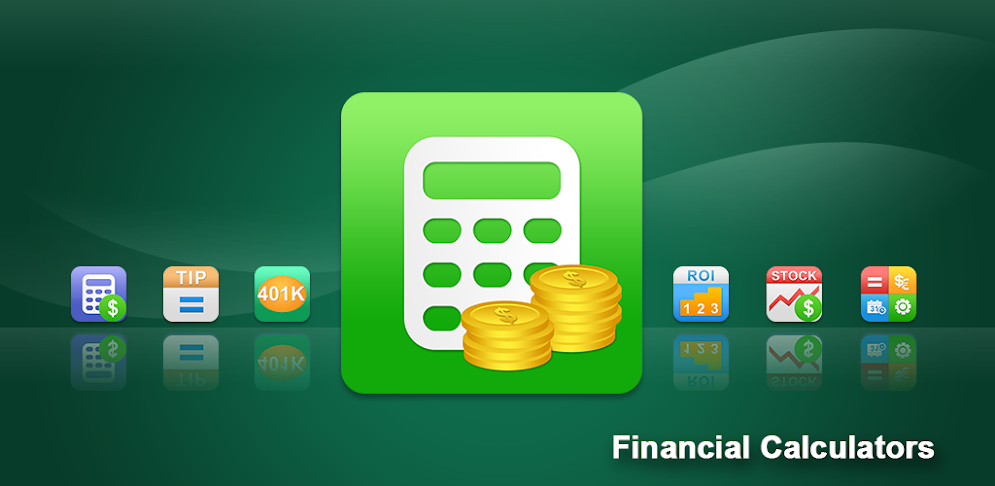## Description

For phone and tablet, this application includes the complete package of financial calculators by Bishinews, a Google rated top developer:

Finance and Investment Calculators

* TVM Calculator
* Currency Converter
* Compound Interest Calculator
* Return On Investment (ROI) Calculator
* IRR NPV Calculator
* MIRR Calculator
* Bond Calculator
* Tax Equivalent Yield Calculator
* Rule of 72 Calculator

Loan/Mortgage Calculators

* Loan Calculator
* Loan Comparison Calculator
* Loan Refinance Calculator
* APR Calculator
* APR Advanced Calculator
* Commercial Loan Calculator
* Simple Loan Calculator
* Loan Analysis Calculator
* Home Affordability Calculator
* Rent vs Buy Calculator
* Mortgage Tax Saving Calculator
* Discount Points Calculator
* Adjustable Rate Calculator
* Fixed vs Adjustable Rate Calculator
* Bi-weekly Payment Calculator
* Interest Only Calculator

Retirement Calculators

* Retirement Planner
* 401k Contribution Calculator
* Retirement Calculator
* Retirement Savings Analysis
* Retirement Income Analysis
* Traditional IRA vs Roth IRA
* Required Minimum Distribution
* Social Security Estimator
* Asset Allocation Calculator
* 401k Save the Max Calculator
* College Savings Calculator

Stock Calculators

* Stock Return Calculator
* Stock Constant Growth Calculator
* Stock Non-constant Growth Calculator
* CAPM Calculator
* Expected Return Calculator
* Holding Period Return Calculator
* Weighted Average Cost of Capital Calculator
* Pivot Point Calculator
* Fibonacci Calculator
* Black-Scholes Option Calculator

Credit Card Calculators

* Credit Card Payoff Calculator
* Credit Card Minimum Calculator

Auto Loan and Lease Calculators

* Auto Loan Calculator
* Auto Lease Calculator

Miscellaneous Calculators

* Regular Calculator
* Tip Calculator
* Discount and Tax Calculator
* Percentage Calculator
* Date Calculator
* Unit Conversion
* US Inflation Calculator
* Margin and Markup Calculator
* Fuel Calculator
* Salary Increase Calculator
* US Paycheck Tax Calculator
* Net Distribution Calculator
* Effective Rate Calculator
* Mutual Fund Fee Calculator
* Unit Price Compare Calculator
* Balance Sheet and Income Statement Analysis
* Financial Ratios

User can send the calculation results to others via Email. Financial Professionals can email the quote to their clients.

The app allows you to edit and prioritize the list of calculators for easy access.

Only Currency Converter needs internet access to retrieve the latest currency exchange rate. The app works without internet connection.

Photos/Media/File Permission: For phones with Android 4.3 and below, this permission is needed for sending email with amortization and chart. For Android system 4.4 and above this permission is not needed any more. You can go to phone Settings/apps/Financial Calculators/Permission to check. You can turn off this permission if you don't use email feature and Android version on your phone is 4.3 and below.

This is pro edition of the popular Financial Calculators app. This app has no Ads.

Please send question and suggestion to us at [email protected] We actively support users.

## Screenshots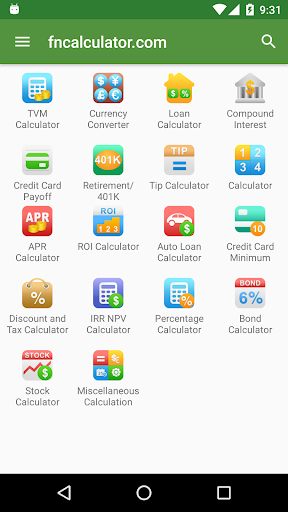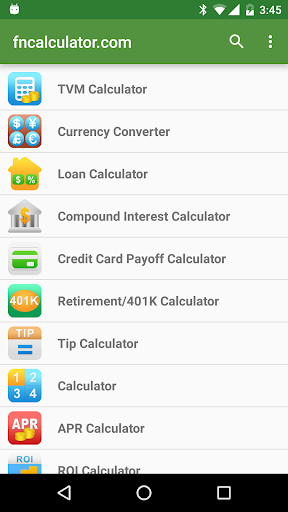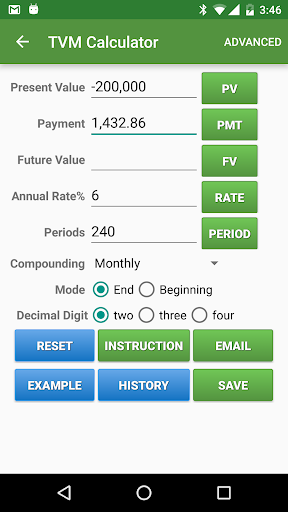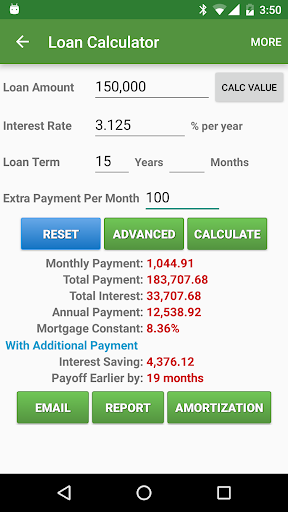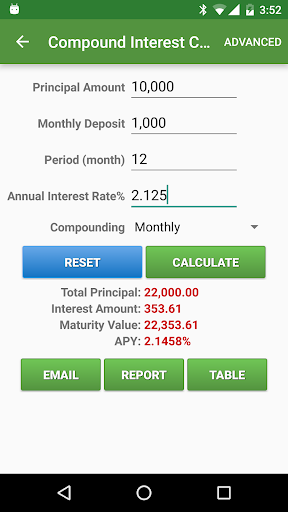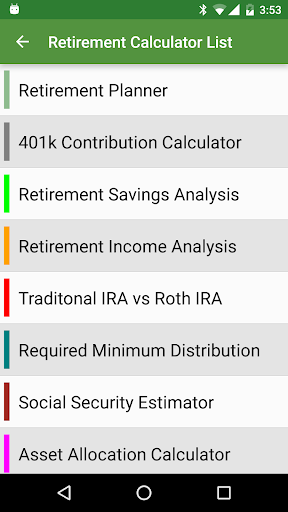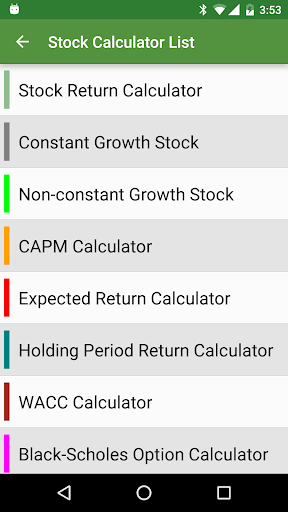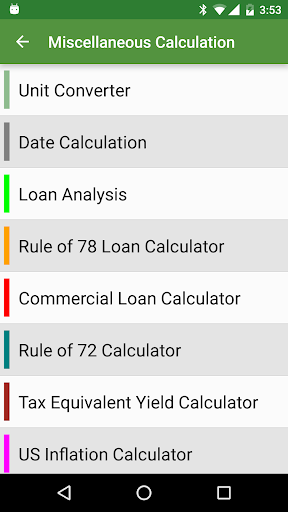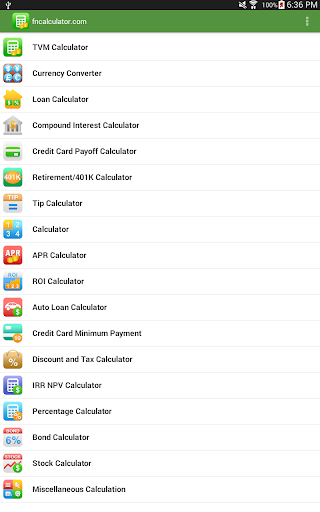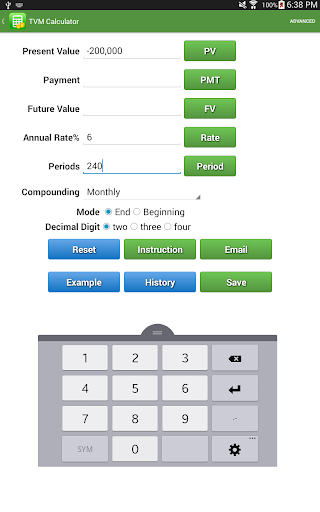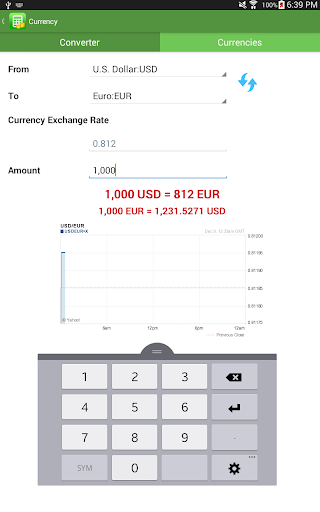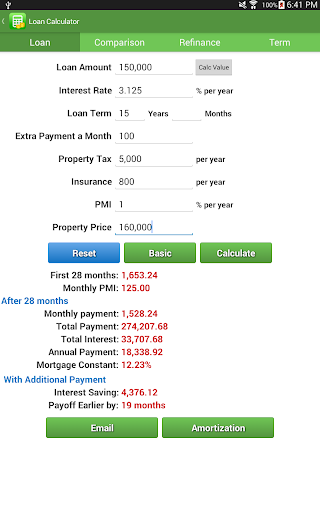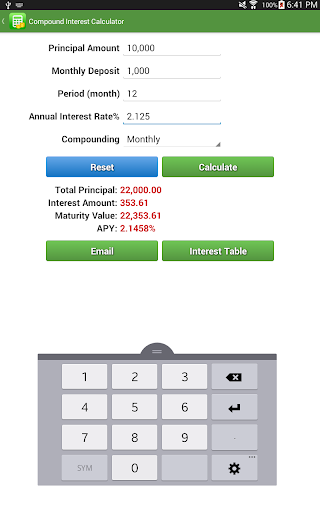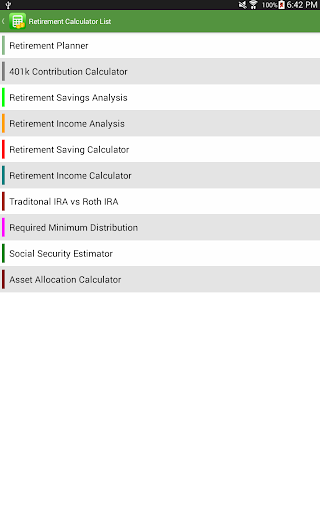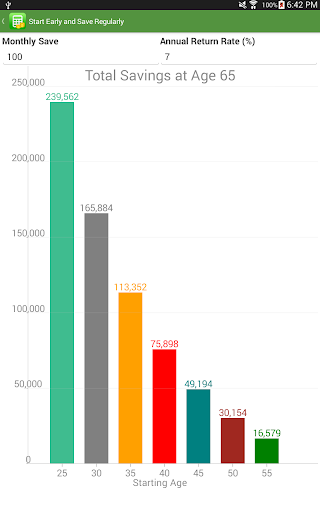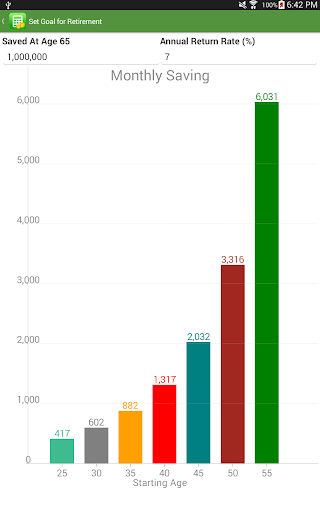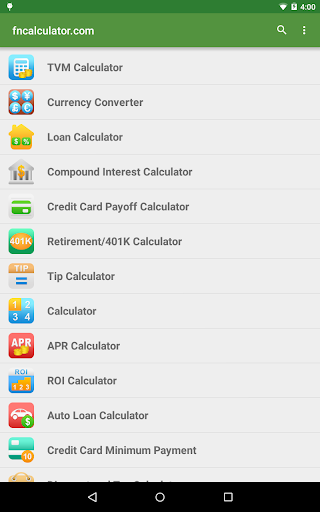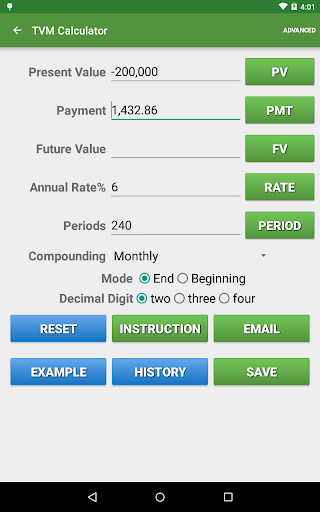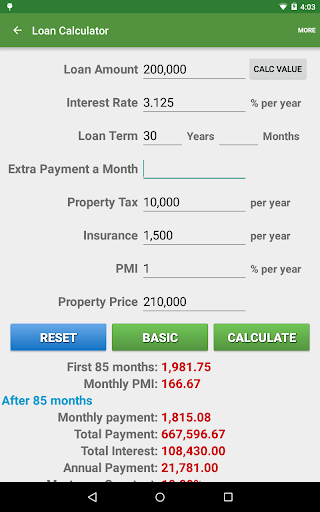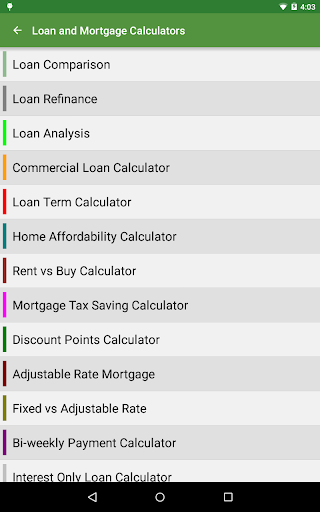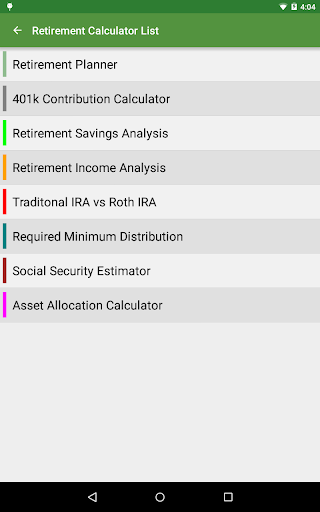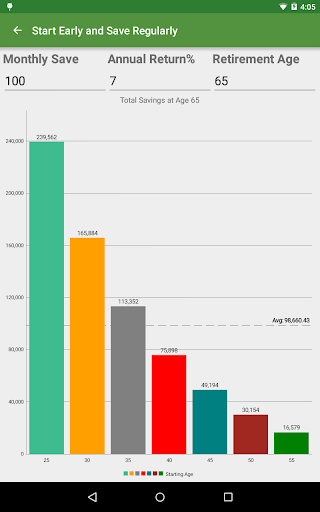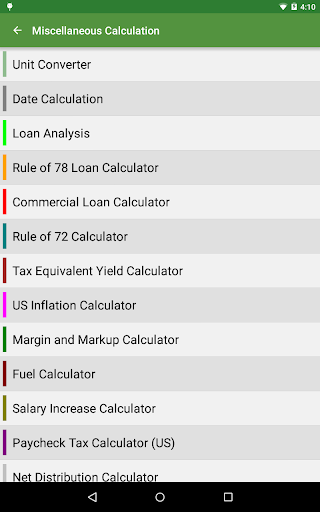Download cracked APK of Financial Calculators Pro ( com.financial.calculator.pro )

 Version 2.9.0 Uploader Hoster clive userscloud.comDownload